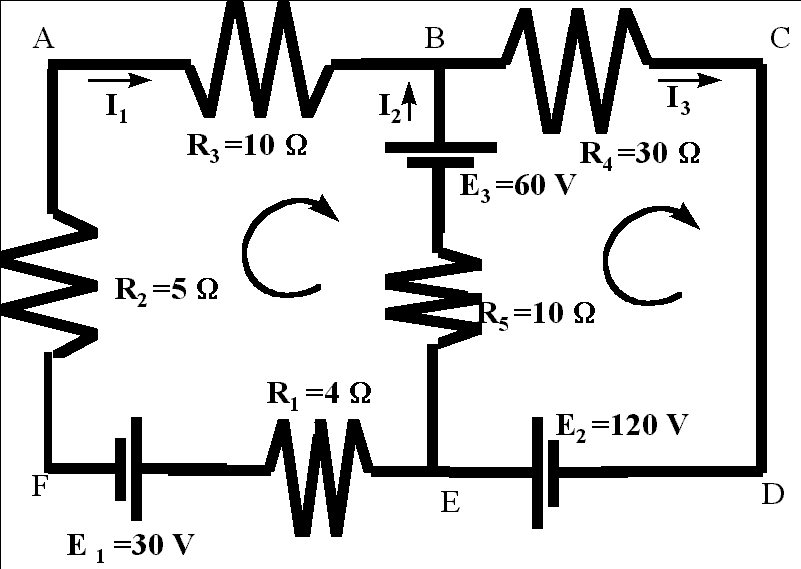Electrical Circuits
A simple Electric Circuit is a closed connection of Batteries , Resistors , Wires. An Electric circuit consist of voltage loops and current nodes .The following physical quantities  are  measured  in an electrical circuit;
Current,: Denoted by  I measured in Amperes (A).
Resistance ,: Denoted by  R  measured in  Ohms ( W ) .
Electrical Potential Difference ,: Denoted by  V  measured in  volts. (v)

Three basic laws govern the flow of current in an electrical circuit :

2. Kirchhoff's Voltage Law Conservation of Energy.

3. Kirchhoff's Current Law Conservation of Charge .

Simple circuits are categorized  in  two type :

For circuits with series and parallel sections, break the circuit up into portions of series and
parallel, then calculate values for these portions, and use these
values to calculate the resistance of the entire circuit. That is,
first,  for each individual series path, calculate the total resistance for that path.
Second, using these values, by assuming that each path as a single resistor, calculate the total resistance of
the circuit.

We can apply  the methods for solving linear systems to solve problems  involving  electrical circuits. In a given circuit if  enough values of currents, resistance, and potential difference  is known, we should be able to find the other unknown values of these quantities. We mainly use the Ohm's Law , Kirchhoff's Voltage Law  and Kirchhoff's current Law.

Example:  Find the currents in the circuit for the following network.Solution : Lets assign currents to each part of the  circuit between the node points.  We have two node points  Which will give us three different currents.  Lets assume that the currents are in clockwise direction.So the current on the segment EFAB is I1, on the segment BCDE is I3 and on the segment EB is I2

Using the  Kirchhoff's current Law for the node  B   yields the equation

I1 + I2 = I3.

For the node E we will get the same equation.Then we use Kirchhoff's  voltage law

-4 I1+ (-30) -5 I - 10I -60  +10I2 =0

When  through the  battery from (-) to (+),   on the segment EF,  potential difference  is  -30, and on segment FA  moving through the resistor of  5W
will result in the potential difference of -5 I and  in a similar way we can find the potential differences on the other segment of the loop EFAB.

In the  loop  BCDE, Kirchhoff's  voltage law will yield the following equation:

-30 I3+ 120-10I2+60 =0

Now we have three equations with three unknowns:

I1          +     I2    -    I3           =0
-19 I +10 I2                     = 90
-10 I2      -30 I3          = -180

This linear system can be solved  by methods of linear Algebra.  Linear Algebra is more useful when the network is very complicated and  the number of the unknowns is large.

The system above has the following solution:

I1   = -1.698
I2 = 5.7736
I=
4.0755

Exercises,